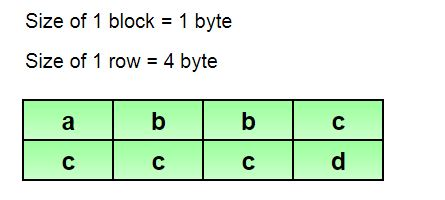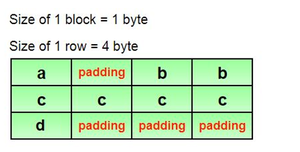# Data Structure alignment

• Difficulty Level : Medium
• Last Updated : 08 Oct, 2021

Data structure alignment is the way data is arranged and accessed in computer memory. Data alignment and Data structure padding are two different issues but are related to each other and together known as Data Structure alignment.
Data alignment: Data alignment means putting the data in memory at address equal to some multiple of the word size. This increases the performance of system due to the way the CPU handles memory.
Data Structure Padding: Now, to align the data, it may be necessary to insert some extra bytes between the end of the last data structure and the start of the next data structure as the data is placed in memory as multiples of fixed word size. This insertion of extra bytes of memory to align the data is called data structure padding.
Consider the structure as shown below:

```struct
{
char a;
short int b;
int c;
char d;
}```

Now we may think that the processor will allocate memory to this structure as shown below:The total memory allocated in this case is 8 bytes. But this never happens as the processor can access memory as fixed word size of 4 bytes. So, the integer variable c can not be allocated memory as shown above. An integer variable requires 4 bytes. The correct way of allocation of memory is shown below for this structure using padding bytes.The processor will require a total of 12 bytes for the above structure to maintain the data alignment.
Look at the below C++ program:

## CPP

 `// CPP program to test``// size of struct``#include ``using` `namespace` `std;` `// first structure``struct` `test1``{``    ``short` `s;``    ``int` `i;``    ``char` `c;``};` `// second structure``struct` `test2``{``    ``int` `i;``    ``char` `c;``    ``short` `s;``};` `// driver program``int` `main()``{``    ``test1 t1;``    ``test2 t2;``    ``cout << ``"size of struct test1 is "` `<< ``sizeof``(t1) << ``"\n"``;``    ``cout << ``"size of struct test2 is "` `<< ``sizeof``(t2) << ``"\n"``;``    ``return` `0;``}`

Output:

```size of struct test1 is 12
size of struct test2 is 8```

For the first structure test1 the short variable takes 2 byte. Now the next variable is int which requires 4 bytes. So, 2 bytes padding is added after short variable. Now, the char variable requires 1 byte but memory will be accessed in word size of 4 byte so 3 byte of padding is added again. So, total 12 bytes of memory is required. We can similarly calculate the padding for the second structure also. Padding for both of the structures is shown below:

```struct test1
{
short s;
// 2 bytes
int i;
// 4 bytes
char c;
// 1 byte
};

struct test2
{
int i;
// 4 bytes
char c;
// 1 byte
short s;
// 2 bytes
};```

Note :You can minimize the size of memory allocated for a structure by sorting members by alignment.
References :
1) https://en.wikipedia.org/wiki/Data_structure_alignment
2) https://stackoverflow.com/a/119134/6942060
This article is contributed by Mandeep Singh. If you like GeeksforGeeks and would like to contribute, you can also write an article using write.geeksforgeeks.org or mail your article to review-team@geeksforgeeks.org. See your article appearing on the GeeksforGeeks main page and help other Geeks.